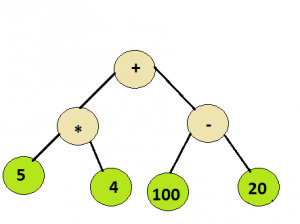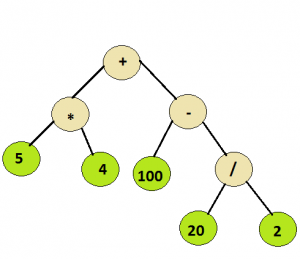Skip to content
Related Articles
Evaluation of Expression Tree
• Difficulty Level : Medium
• Last Updated : 13 Apr, 2021

Given a simple expression tree, consisting of basic binary operators i.e., + , – ,* and / and some integers, evaluate the expression tree.

Examples:

```
Input :
Root node of the below treeOutput :
100

Input :
Root node of the below treeOutput :
110
```

## Recommended: Please solve it on “PRACTICE” first, before moving on to the solution.

As all the operators in the tree are binary hence each node will have either 0 or 2 children. As it can be inferred from the examples above , the integer values would appear at the leaf nodes , while the interior nodes represent the operators.
To evaluate the syntax tree , a recursive approach can be followed .

```Algorithm :
Let t be the syntax tree
If  t is not null then
If t.info is operand then
Return  t.info
Else
A = solve(t.left)
B = solve(t.right)
return A operator B
where operator is the info contained in t

```

The time complexity would be O(n), as each node is visited once. Below is a C++ program for the same:

## C/C++

 `// C++ program to evaluate an expression tree ``#include  ``using` `namespace` `std; `` ` `// Class to represent the nodes of syntax tree ``class` `node ``{ ``public``: ``    ``string info; ``    ``node *left = NULL, *right = NULL; ``    ``node(string x) ``    ``{ ``        ``info = x; ``    ``} ``}; `` ` `// Utility function to return the integer value ``// of a given string ``int` `toInt(string s) ``{ ``    ``int` `num = 0; ``       ` `      ``// Check if the integral value is ``      ``// negative or not``      ``// If it is not negative, generate the number ``      ``// normally``      ``if``(s!=``'-'``)``        ``for` `(``int` `i=0; ileft && !root->right) ``        ``return` `toInt(root->info); `` ` `    ``// Evaluate left subtree ``    ``int` `l_val = eval(root->left); `` ` `    ``// Evaluate right subtree ``    ``int` `r_val = eval(root->right); `` ` `    ``// Check which operator to apply ``    ``if` `(root->info==``"+"``) ``        ``return` `l_val+r_val; `` ` `    ``if` `(root->info==``"-"``) ``        ``return` `l_val-r_val; `` ` `    ``if` `(root->info==``"*"``) ``        ``return` `l_val*r_val; `` ` `    ``return` `l_val/r_val; ``} `` ` `//driver function to check the above program ``int` `main() ``{ ``    ``// create a syntax tree ``    ``node *root = ``new` `node(``"+"``); ``    ``root->left = ``new` `node(``"*"``); ``    ``root->left->left = ``new` `node(``"5"``); ``    ``root->left->right = ``new` `node(``"-4"``); ``    ``root->right = ``new` `node(``"-"``); ``    ``root->right->left = ``new` `node(``"100"``); ``    ``root->right->right = ``new` `node(``"20"``); ``    ``cout << eval(root) << endl; `` ` `    ``delete``(root); `` ` `    ``root = ``new` `node(``"+"``); ``    ``root->left = ``new` `node(``"*"``); ``    ``root->left->left = ``new` `node(``"5"``); ``    ``root->left->right = ``new` `node(``"4"``); ``    ``root->right = ``new` `node(``"-"``); ``    ``root->right->left = ``new` `node(``"100"``); ``    ``root->right->right = ``new` `node(``"/"``); ``    ``root->right->right->left = ``new` `node(``"20"``); ``    ``root->right->right->right = ``new` `node(``"2"``); `` ` `    ``cout << eval(root); ``    ``return` `0; ``} `

## Python

 `# Python program to evaluate expression tree`` ` `# Class to represent the nodes of syntax tree``class` `node:``    ``def` `__init__(``self``, value):``        ``self``.left ``=` `None``        ``self``.data ``=` `value``        ``self``.right ``=` `None`` ` `# This function receives a node of the syntax tree``# and recursively evaluate it``def` `evaluateExpressionTree(root):`` ` `    ``# empty tree``    ``if` `root ``is` `None``:``        ``return` `0`` ` `    ``# leaf node``    ``if` `root.left ``is` `None` `and` `root.right ``is` `None``:``        ``return` `int``(root.data)`` ` `    ``# evaluate left tree``    ``left_sum ``=` `evaluateExpressionTree(root.left)`` ` `    ``# evaluate right tree``    ``right_sum ``=` `evaluateExpressionTree(root.right)`` ` `    ``# check which operation to apply``    ``if` `root.data ``=``=` `'+'``:``        ``return` `left_sum ``+` `right_sum``     ` `    ``elif` `root.data ``=``=` `'-'``:``        ``return` `left_sum ``-` `right_sum``     ` `    ``elif` `root.data ``=``=` `'*'``:``        ``return` `left_sum ``*` `right_sum``     ` `    ``else``:``        ``return` `left_sum ``/` `right_sum`` ` `# Driver function to test above problem``if` `__name__``=``=``'__main__'``:``     ` `    ``# creating a sample tree``    ``root ``=` `node(``'+'``)``    ``root.left ``=` `node(``'*'``)``    ``root.left.left ``=` `node(``'5'``)``    ``root.left.right ``=` `node(``'4'``)``    ``root.right ``=` `node(``'-'``)``    ``root.right.left ``=` `node(``'100'``)``    ``root.right.right ``=` `node(``'20'``)``    ``print` `evaluateExpressionTree(root)`` ` `    ``root ``=` `None`` ` `    ``#creating a sample tree``    ``root ``=` `node(``'+'``)``    ``root.left ``=` `node(``'*'``)``    ``root.left.left ``=` `node(``'5'``)``    ``root.left.right ``=` `node(``'4'``)``    ``root.right ``=` `node(``'-'``)``    ``root.right.left ``=` `node(``'100'``)``    ``root.right.right ``=` `node(``'/'``)``    ``root.right.right.left ``=` `node(``'20'``)``    ``root.right.right.right ``=` `node(``'2'``)``    ``print` `evaluateExpressionTree(root)`` ` `# This code is contributed by Harshit Sidhwa`

Output:
```60
110
```

This article is contributed by Ashutosh Kumar. If you like GeeksforGeeks and would like to contribute, you can also write an article using contribute.geeksforgeeks.org or mail your article to contribute@geeksforgeeks.org. See your article appearing on the GeeksforGeeks main page and help other Geeks.

Please write comments if you find anything incorrect, or you want to share more information about the topic discussed above.

Attention reader! Don’t stop learning now. Get hold of all the important DSA concepts with the DSA Self Paced Course at a student-friendly price and become industry ready.  To complete your preparation from learning a language to DS Algo and many more,  please refer Complete Interview Preparation Course.

In case you wish to attend live classes with industry experts, please refer Geeks Classes Live

My Personal Notes arrow_drop_up